• 介绍两种最简单实用的方法，方法如下：一、简介(1)Roberts 算子 基于 Roberts算子的边缘检测法是通过局部差分算法对图像进行运算处理，进而得到图像边界点的检测方法，其边界点的检测公式如下：这个公式的意思是...
介绍两种最简单实用的方法，方法如下：一、简介(1)Roberts 算子   基于 Roberts算子的边缘检测法是通过局部差分算法对图像进行运算处理，进而得到图像边界点的检测方法，其边界点的检测公式如下：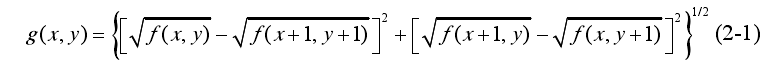这个公式的意思是，当边缘不存在的时候，图形中边缘内部会全部变为0，只有边缘才会变成1，最终我们得到的图像就是边缘图像。(2) Sobel 算子      基于 Sobel 算子的边缘检测法由包含水平边缘和垂直边缘信息的卷积核组成，其中水平边缘信息的卷积核对该检测法的影响较大。该方法的主要原理是通过卷积算法得到水平边缘和垂直边缘的最大值，将该值作为 Sobel 算子输出，进而实现对图像的边缘检测并提取。 二、matlab仿真#读入图象：a=imread(2.jpg);subplot(3,1,1);imshow(a);#采用Roberts算子b=edge(rgb2gray(a));subplot(3,1,2);imshow(b);#Sobel算子：c=edge(rgb2gray(a)); subplot(3,1,2);imshow(c);效果图如下，可见，边缘被很好的检测了出来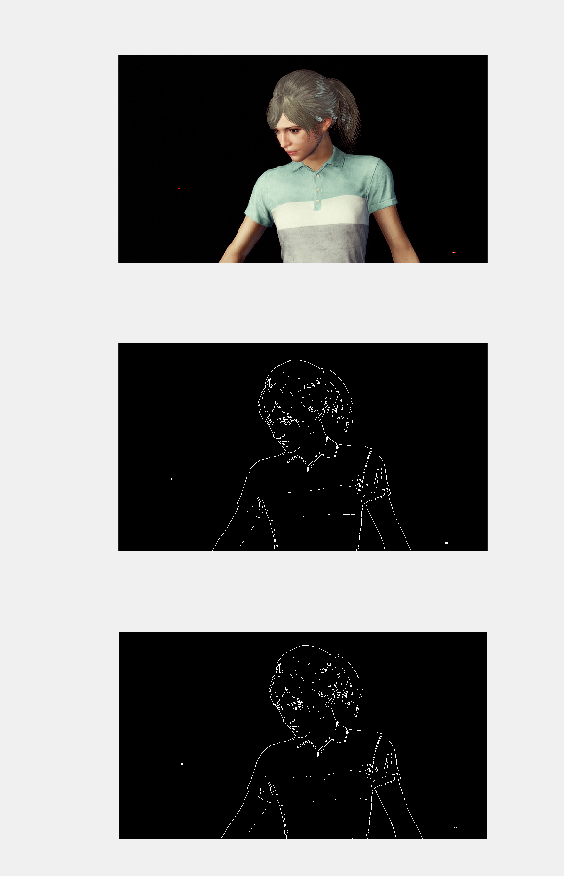展开全文图像处理
• 使用MATLAB对彩色图像进行边缘检测的方法的讨论
截止到本文写作时，据了解，MATLAB R2017B中尚无针对彩色图像进行边缘检测的函数，因此，笔者在课程设计的压力下思考了这个问题，并将其整理为文字，以供分享
本文所用软件为MATLAB R2017B，经测试，稳中提供代码可在MATLAB R2016A上完美运行，更低版本未测试
实验中采用Laplace算子进行边缘检测；这张图片为这次试验中使用的原图，可以随意下载使用方法一 将彩色图转换为灰度图再进行边缘检测

这种方法的缺点是相比于方法二来说，会损失更多信息量，具体代码如下
I_origin2 = imread('img3.jpg');

I_gray = rgb2gray(I_origin2);

Edge_gray = edge(I_gray, 'log');

产生的效果图：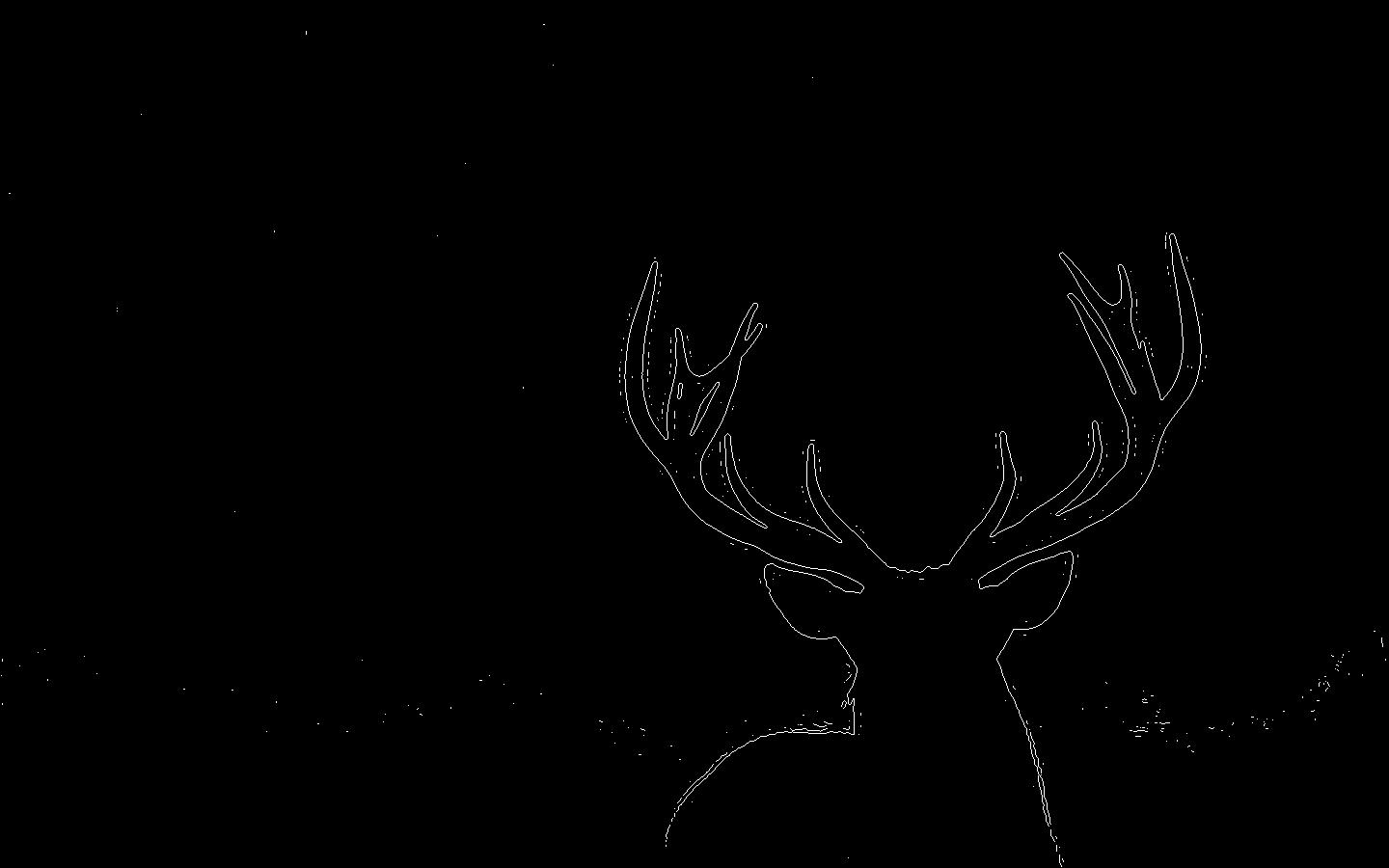方法二 提取彩色图像的RGB分量，分别进行边缘检测后再进行叠加合成

a. 提取RGB分量
Instance_R = I_origin2(:, :, 1);
Instance_G = I_origin2(:, :, 2);
Instance_B = I_origin2(:, :, 3);

效果图：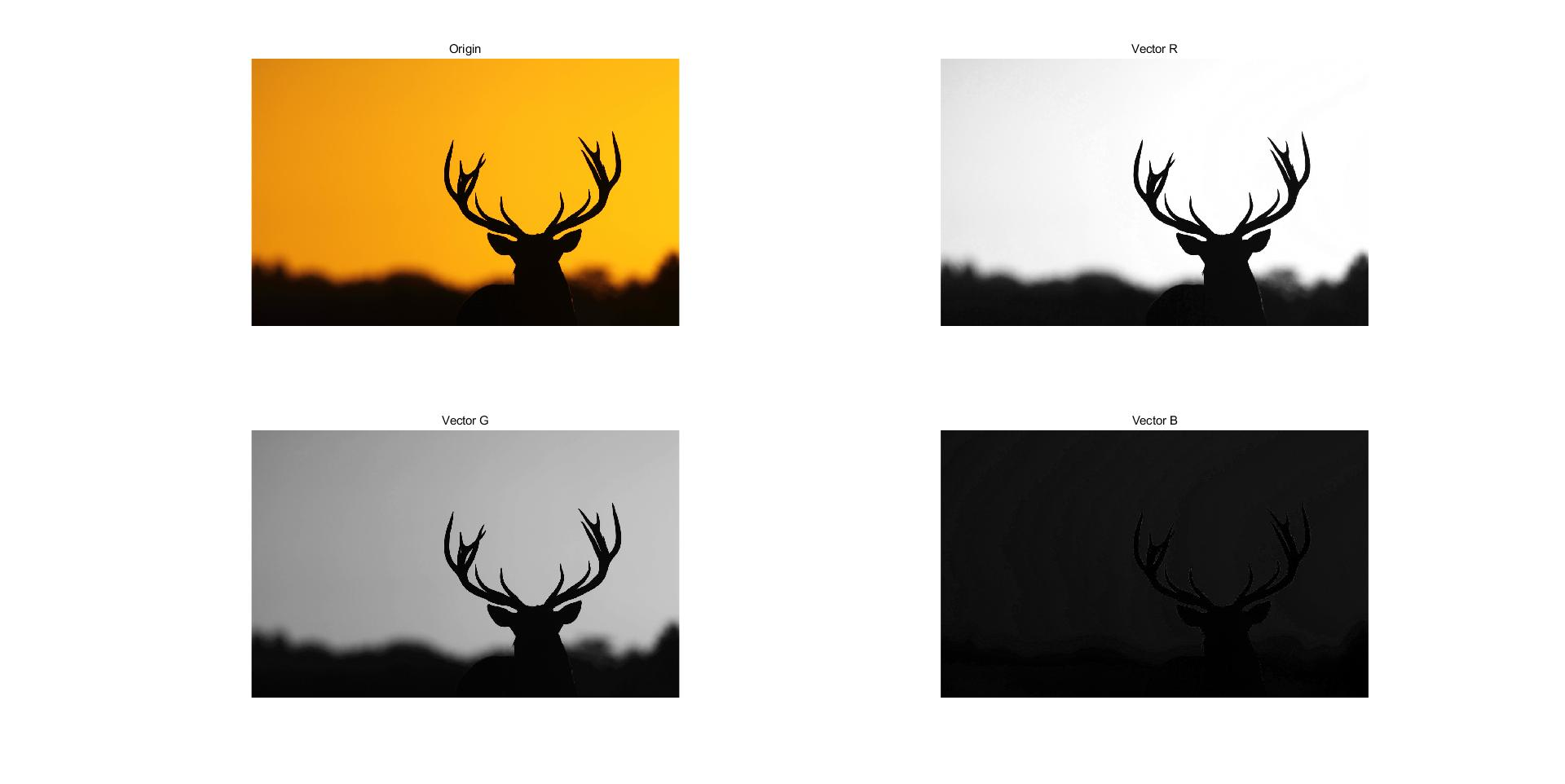b. 对RGB分量分别进行边缘检测


Edge_R = edge(Instance_R, 'log');
Edge_G = edge(Instance_G, 'log');
Edge_B = edge(Instance_B, 'log');

效果图：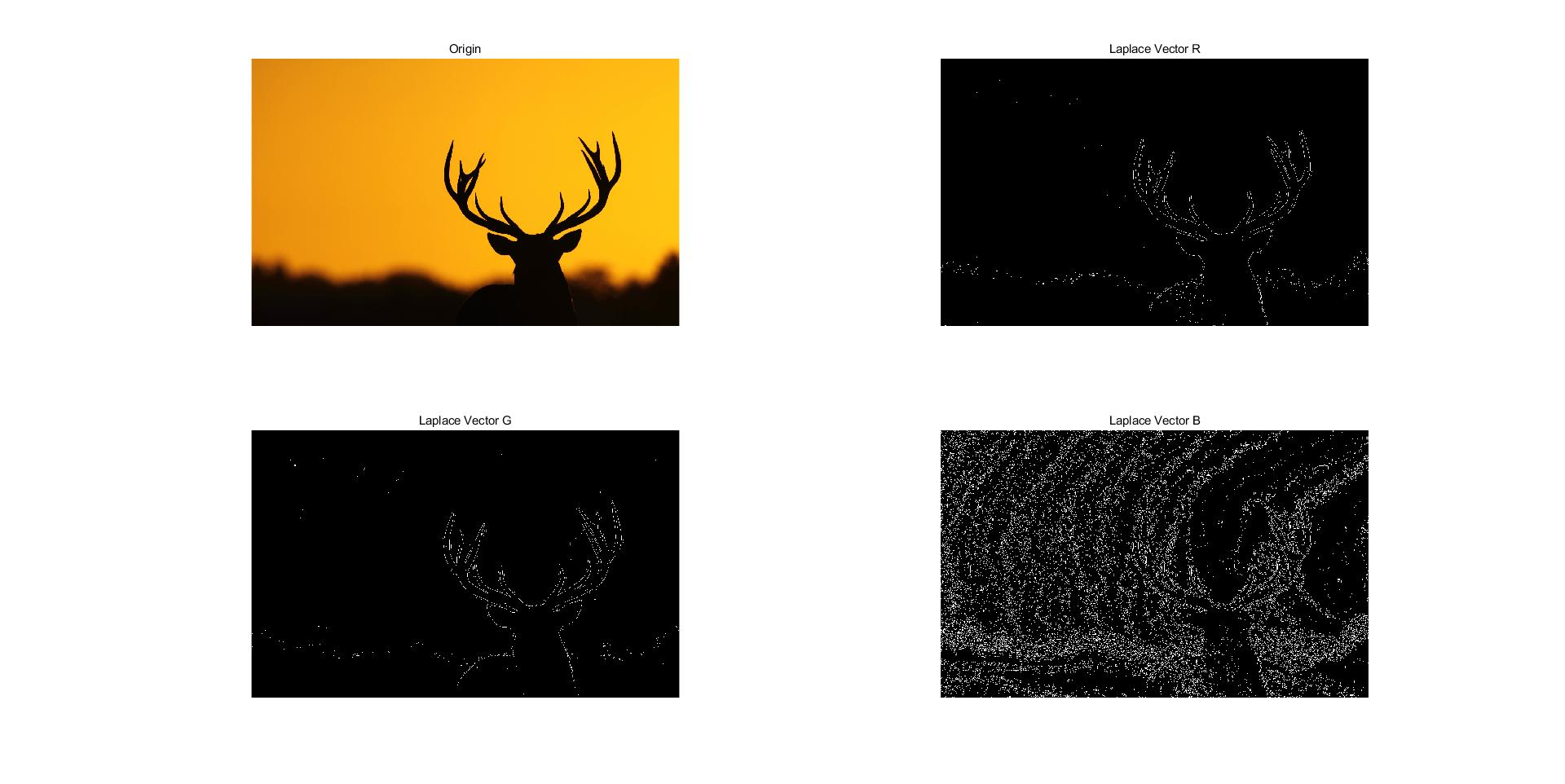c. 对上一步结果进行叠加合成

rgb = im2uint8(cat(3, Edge_R, Edge_G, Edge_B));

效果图：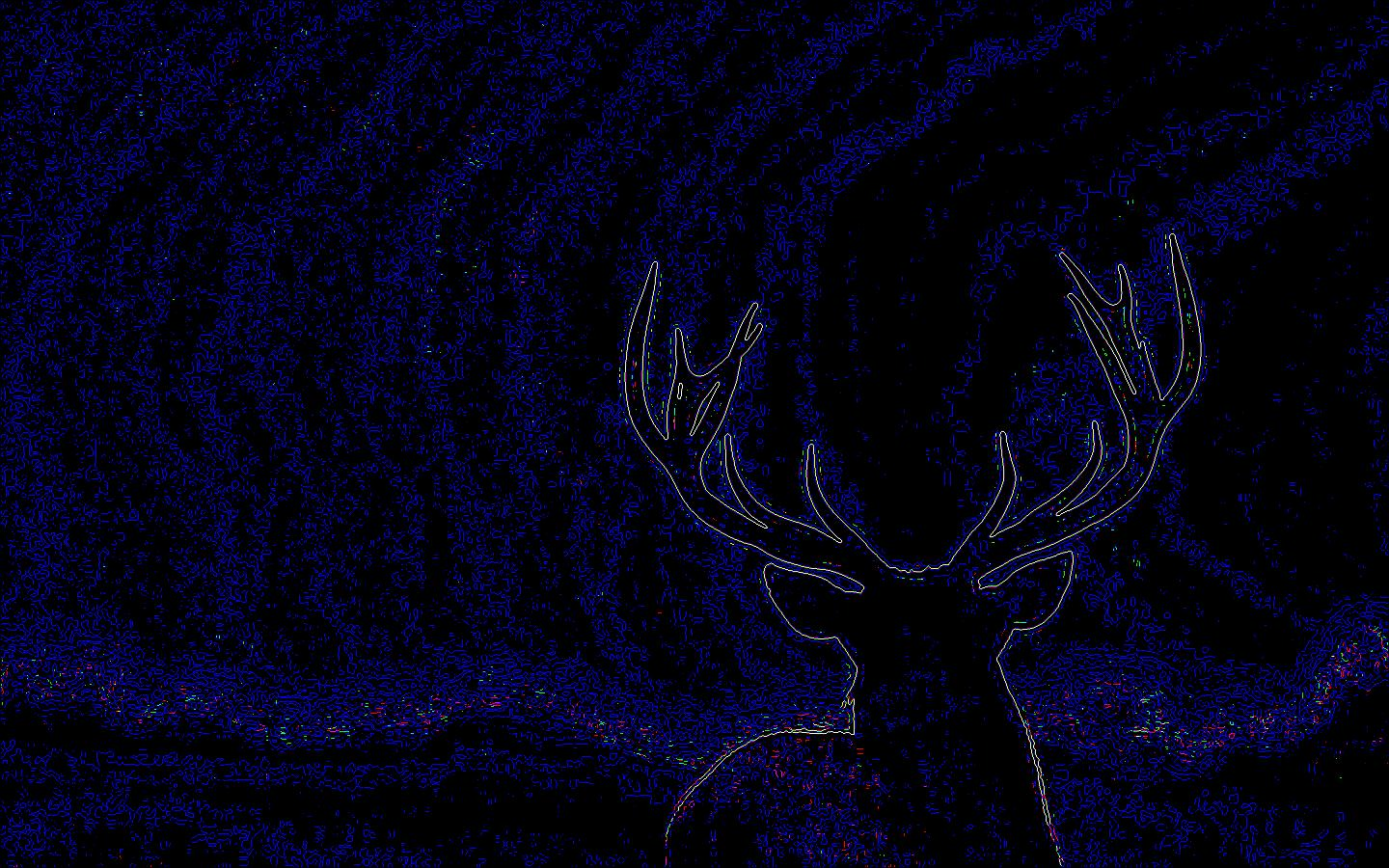可以看到，在这张图中，不论是鹿的轮廓还是鹿背后起伏的地形，其形状都得到了很好的保留，然而美中不足的是，这张图片中有很多的蓝色噪点，这些也是需要通过某种方法消除的，最简单的方法就是将该图片进行一次灰度图的转换
d. 对此方法的改进 
I_RGB = rgb2gray(rgb); 
效果图：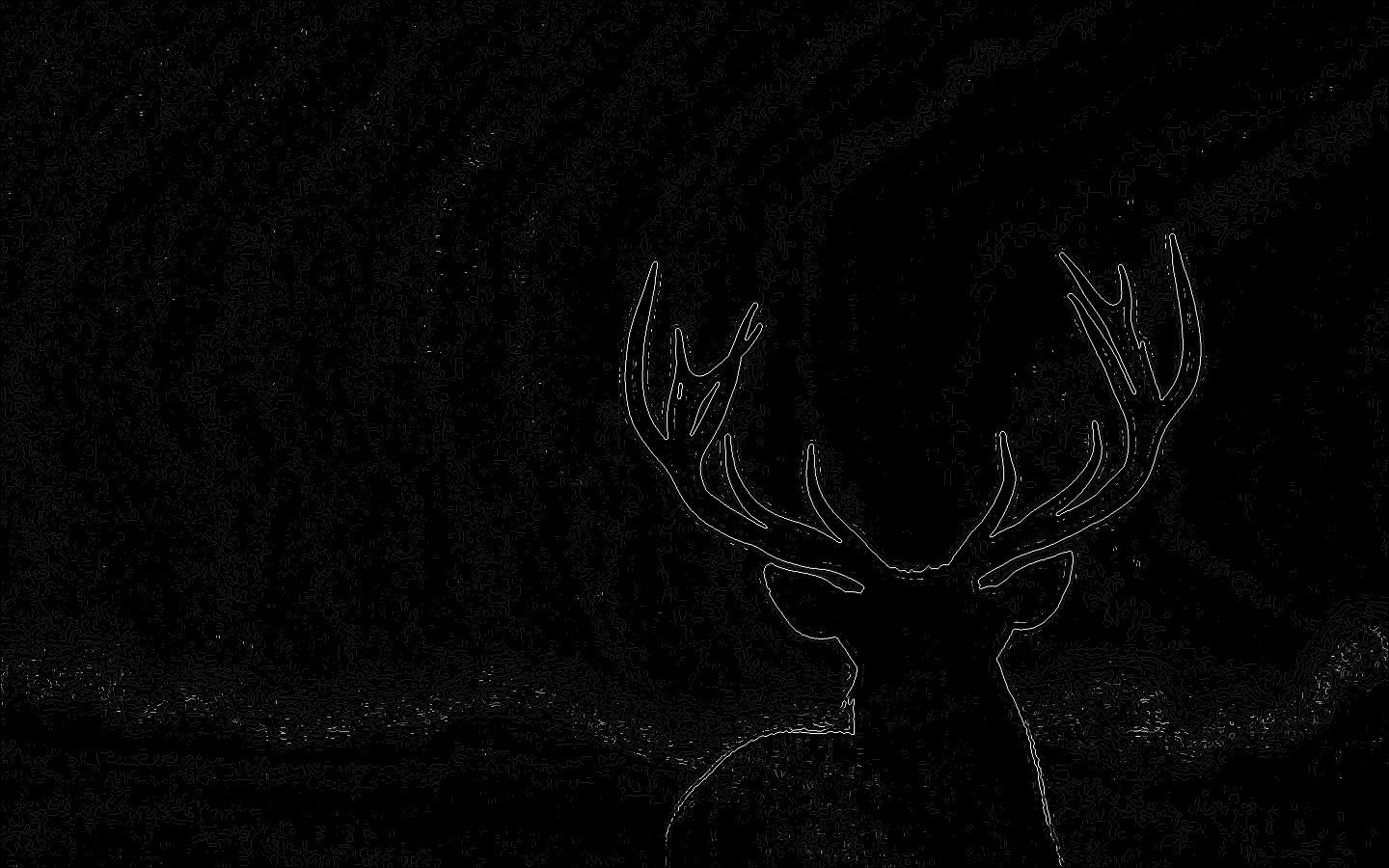可以看到，这张图片的效果有了明显的改善，噪点的数量下降很多，边缘相对于第一种方法也更加清晰。因此这个效果是比较理想的

完整的源代码

这次实验一共三个源文件，分别贴在下面：
    % image_edge.m
% 探究对真彩色图像的边缘检测方法
% ----两种边缘检测方法(均以Laplace算子为例)
% -----------------1. 真彩色图像->灰度图->边缘检测
% -----------------2. 真彩色图像->分解为RGB分量->对分量进行边缘转换->对转换结果进行合并->剔除不合适的分量

%初始化运行环境
close all; clear; clc;
warning off;

% 读取图像并适当进行压缩
I_origin = imread('img3.jpg');

[size_x, size_y, size_z] = size(I_origin);
if size_x > 1080
I_origin2 = imresize(I_origin, 1080 / double(size_x));
else
I_origin2 = I_origin;
end

figure(1), imshow(I_origin2), title('原图');

% ---- 方法一
I_gray = rgb2gray(I_origin2);
figure('Name', '对灰度图的边缘检测');
subplot(1, 2, 1), imshow(I_origin2), title('原图');
subplot(1, 2, 2), imshow(I_gray), title('灰度图');

Edge_gray = edge(I_gray, 'log');

% ---- 方法二
% ---------------提取RGB分量并显示
Instance_R = I_origin2(:, :, 1);
Instance_G = I_origin2(:, :, 2);
Instance_B = I_origin2(:, :, 3);
figure('Name', '原图的RGB分量');
subplot(2, 2, 1), imshow(I_origin2), title('Origin');
subplot(2, 2, 2), imshow(Instance_R), title('Vector R');
subplot(2, 2, 3), imshow(Instance_G), title('Vector G');
subplot(2, 2, 4), imshow(Instance_B), title('Vector B');

% ---------------对RGB分量进行边缘检测并合并
Edge_R = edge(Instance_R, 'log');
Edge_G = edge(Instance_G, 'log');
Edge_B = edge(Instance_B, 'log');
rgb = im2uint8(cat(3, Edge_R, Edge_G, Edge_B));

figure('Name', 'RGB分量的边缘检测');
subplot(2, 2, 1), imshow(I_origin2), title('Origin');
subplot(2, 2, 2), imshow(Edge_R), title('Laplace Vector R');
subplot(2, 2, 3), imshow(Edge_G), title('Laplace Vector G');
subplot(2, 2, 4), imshow(Edge_B), title('Laplace Vector B');

figure('Name', '两种检测方法的对比');
subplot(1, 2, 1), imshow(Edge_gray), title('方法一');
subplot(1, 2, 2), imshow(rgb), title('方法二');

%EOF


    % image_sub1.m
% 探究对真彩色图像的边缘检测方法
% ----两种边缘检测方法(均以Laplace算子为例)

% ！！！所有sub文件均需在image_edge.m执行后执行！！！

% 灰度图的边缘检测与彩色图分别除去RGB分量的边缘检测对比
figure('Name', 'image_sub1');
subplot(2, 2, 1), imshow(Edge_gray), title('Gray');
subplot(2, 2, 2), imshow(cat(3, zeros(size(Edge_R)), Edge_G, Edge_B)), title('Without R');
subplot(2, 2, 3), imshow(cat(3, Edge_R, zeros(size(Edge_G)), Edge_B)), title('Without G');
subplot(2 ,2, 4), imshow(cat(3, Edge_R, Edge_G, zeros(size(Edge_B)))), title('Without B');

% EOF


    % image_sub2.m
% 探究对真彩色图像的边缘检测方法
% ----两种边缘检测方法(均以Laplace算子为例)

% ！！！所有sub文件均需在image_edge.m执行后执行！！！

% 对彩色图执行RGB边缘检测后取灰度化与灰度化边缘检测对比
figure('Name', 'image_sub2');
subplot(1, 2, 1), imshow(Edge_gray), title('Gray');
subplot(1, 2, 2), imshow(rgb2gray(rgb)), title('RGB to Gray');

%EOF

对于此实验的一些思考
以目前的测试结果看，这份代码非常适合有大片色块且色块色差比较大的图片，而对于一些整体色调一致的图片而言，方法一二区别不大，但是二依然存有微弱优势
对此提出一种尚未证实的猜想，即对于色调几乎保持一致的图片，对RGB分量进行边缘检测后，舍弃效果较差的分量，再进行叠加合成并灰度化，效果应该比方法二要好一些，现在不知道是不是可以对RGB分量的强度进行微调而不是直接舍弃，如果可以分别调整RGB分量的话，配合平滑滤波，效果应该会更好。
这个猜想笔者会进行测试后分享到博客，如果对这篇文章有什么疑问或者批评指正，请在评论区留言~_~

展开全文图片 数字图像处理
• 简要介绍了边缘检测技术，结合实例详细分析了MATLAB在图像边缘检测中的具体应用方法图像处理
• 学习过程遇到了不少不懂东西，好在自学能力还可以（自恋= =），所以断断续续也算学会了一些东西~因为这段时间一直在做边缘检测和提取工作，所以本篇就总结一下一些常见的边缘检测方法，篇幅较长，可按点...
写在前面
呼~最近开始入坑图像+机器学习了，学习的过程中遇到了不少不懂的东西，好在自学能力还可以（自恋中= =），所以断断续续也算学会了一些东西因为这段时间一直在做边缘检测和提取的工作，所以本篇就总结一下一些常见的边缘检测方法，篇幅较长，可按点查看
名词解释
图像处理中经常用到一些名词，以下列举一些：
1. 滤波
所谓滤波就是对每个像素点及其邻域点的灰度值按照一定的参数规则进行加权平均，这样可以有效滤去理想图像中叠加的高频噪声。常用的滤波有线性滤波、中值滤波、均值滤波、双边滤波、高斯滤波等。滤波有抑制噪声的作用，但这会使得图像边缘模糊。
2. 直方图
在图像处理中，经常用到直方图，如颜色直方图、灰度直方图等。直方图可以直观展现数据分布情况，如灰度直方图中，横坐标为各个灰度范围，纵坐标为处在相应范围的像素数。图像直方图不关心像素所处的空间位置，因此不受图像旋转和平移变化的影响，可以作为图像的特征。
3. 上采样
上采样即放大图像（或称图像插值（interpolating））,从而使图像可以显示在更高分辨率的显示设备上。注意对图像的缩放操作通常会影响图像的质量。上采样几乎都是采用内插值方法，即在原有图像像素的基础上在像素点之间采用合适的插值算法插入新的元素。
4. 下采样
下采样即缩小图像（或称为降采样（downsampled）），其主要目的有两个：1、使得图像符合显示区域的大小；2、生成对应图像的缩略图。
下采样原理：对于一幅图像I尺寸为M*N，对其进行s倍下采样，即得到(M/s)*(N/s)尺寸的分辨率图像，当然s应该是M和N的公约数才行，如果考虑的是矩阵形式的图像，就是把原始图像s*s窗口内的图像变成一个像素，这个像素点的值就是窗口内所有像素的均值
Canny算子
1.原理
canny算子与LoG算子类似，属于先平滑后求导数的方法，原理可分成下面4个部分：
1.1 高斯滤波
在所有滤波方法中，需要考虑的最重要的一点是如何平衡去噪与边缘检测精确之间的矛盾。实际工程经验表明，高斯函数确定的核可以提供较好的折衷方案。
高斯滤波实现方法有两种：离散化窗口滑动卷积、傅里叶变换。因前者比较常用，故下面只介绍前者。
离散化窗口滑动卷积主要利用高斯核实现，即一个奇数大小的高斯模板。常用的高斯核模板有3*3 和 5*5两种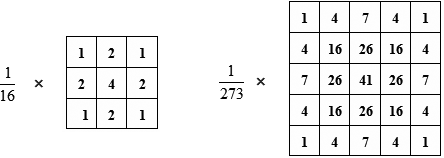其中的参数通过高斯函数计算，x2+y2表示像素点和中心像素点的距离，sigma表示标准差。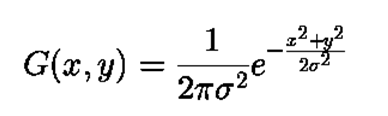注：
sigma如果选的过大，会加深滤波程度，从而导致图像边缘模糊，不利于下一步的边缘检测，如果过小，则滤波效果不佳
计算高斯模板参数时，需要归一化处理，对于归一化的原因，有一种解释是：归一化之后，通过卷积计算出来的模板中心像素被限制到了0-255的灰度区间中。假若某一邻域内所有像素的灰度值为255，利用该模板进行卷积之后，求得的模板中心像素灰度值仍然为255；假若计算出来的高斯模板参数之和小于1，那么通过该模板进行卷积之后，模板中心像素的灰度值将小于255，偏离了实际的灰度值，产生了误差。

1.2 求梯度幅值和梯度方向
canny算子使用的卷积算子如下：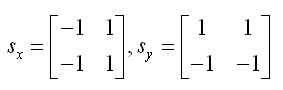梯度幅值及梯度方向的计算如下，其中P表示x方向一阶偏导数矩阵，Q表示y方向一阶偏导数矩阵，M表示梯度幅值，θ表示梯度方向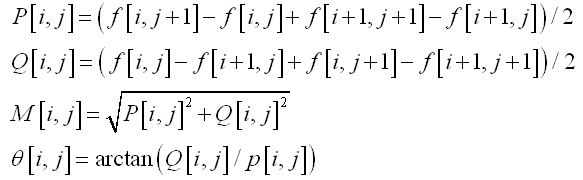1.3 非极大值抑制
在上面求出的梯度幅值矩阵中，值越大的元素代表其梯度越大，但它不一定是边缘像素，因此需要进行非极大值抑制，也就是寻找像素点局部最大值，将非极大值点所对应的灰度值置为0，这样可以剔除掉一大部分非边缘的点。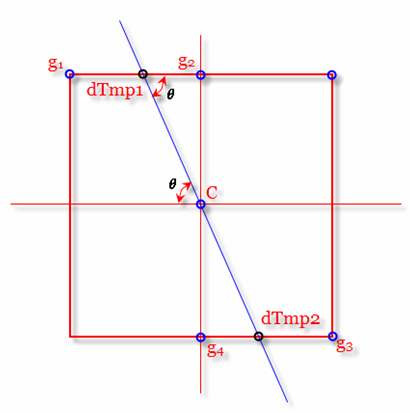如上图，判定C点是否为8邻域内最大梯度值点，只需要判断C是否比C的梯度方向上dTmp1和dTmp2点大，是则保留，不是则将C点灰度值置0。而dTmp1和dTmp2的梯度值可以通过插值得到。
1.4 双阈值法闭合边缘
上面得到的边缘有些为假边缘，且有边缘断裂的问题，如果根据高阈值得到一个边缘图像，这样一个图像含有很少的假边缘，但是由于阈值较高，产生的图像边缘可能不闭合，因此还要采用一个低阈值，当到达轮廓的端点时，在断点的8邻域点中寻找满足低阈值的点，再根据此点收集新的边缘，直到整个图像边缘闭合。
2.实现
2.1 原始图像灰度化
img = imread('lena.jpg');
img = rgb2gray(img);

2.2 调用matlab内置函数
img_edge = edge(img,'canny');
figure;imshow(img_edge);title('canny');

Roberts、Sobel、Prewitt算子
图像的灰度值梯度可以用一阶偏导的有限差分近似计算，常用梯度算子有：
Roberts算子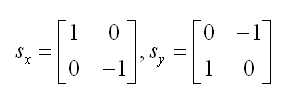梯度幅值为：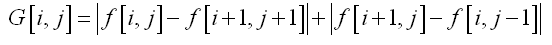matlab中可直接调用：edge(img,'roberts')
Sobel算子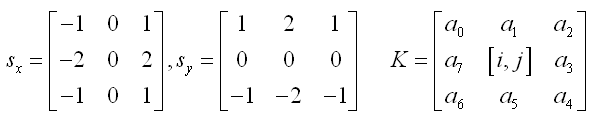梯度幅值为：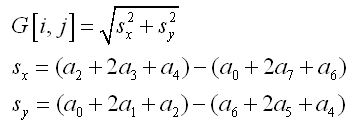matlab中可直接调用：edge(img,'sobel')
Prewitt算子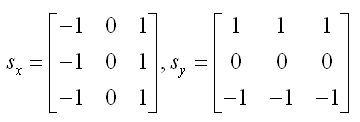matlab中可直接调用：edge(img,'prewitt')
双边滤波（Bilateral Filters）
1. 原理
传统滤波方法多多少少会有模糊边缘的缺点，而双边滤波作为一种非线性滤波器，具有在降噪平滑的同时，保持边缘的效果。该特性主要是通过在卷积的过程中组合空域(space)函数和值域(range)核函数来实现的，空域指的是像素的欧氏距离，值域指的是像素范围域中的辐射差异（如卷积核中像素与中心像素之间相似程度、颜色强度，深度距离等）。典型的核函数为高斯分布函数，如下所示：
[外链图片转存失败(img-nOZjYtOC-1562302602305)(https://img-my.csdn.net/uploads/201205/30/1338365238_1668.jpg)]
其中，权重系数w(i,j,k,l)取决于空域核和值域核的乘积：
[外链图片转存失败(img-gjxNA9ai-1562302602306)(https://img-my.csdn.net/uploads/201205/30/1338365512_2777.jpg)]
在图像的平坦区域，像素值变化很小，对应的像素值域权重接近于1，此时空域权重起主要作用，相当于进行高斯模糊；在图像的边缘区域，像素值变化很大，像素值域权重变大，从而保持了边缘的信息。
2. 实现
上述方法的时间复杂度是O(σd^2)，非常耗时。论文《Fast O(1) bilateral ﬁltering using trigonometric range kernels》，提出了用Raised cosines函数来逼近高斯值域函数，并利用一些特性把值域函数分解为一些列函数的叠加，从而实现函数的加速，而论文" A fast approximation of the bilateral filter using a signal processing approach"则提出了一种使用信号处理的方法，主要是在原有域上添加了信号强度这一维，构成了高维空间，在高维空间中进行下采样，下面的代码是作者团队编写的：
% 双边滤波函数
function output = fBilateralFilter_ReviseVer( data, edge, edgeMin, edgeMax, sigmaSpatial, sigmaRange,samplingSpatial, samplingRange )
if ~exist( 'edge', 'var' )
edge = data;
elseif isempty( edge )
edge = data;
end

inputHeight = size( data, 1 );
inputWidth = size( data, 2 );

if ~exist( 'edgeMin', 'var' )
edgeMin = min( edge( : ) );
% 	warning( 'edgeMin not set!  Defaulting to: %f\n', edgeMin );
end

if ~exist( 'edgeMax', 'var' )
edgeMax = max( edge( : ) );
% 	warning( 'edgeMax not set!  Defaulting to: %f\n', edgeMax );
end

edgeDelta = edgeMax - edgeMin;% hl- span of range

% hl- assign scale parameters in both spatial and range domain
if ~exist( 'sigmaSpatial', 'var' )
sigmaSpatial = min( inputWidth, inputHeight ) / 16;
fprintf( 'Using default sigmaSpatial of: %f\n', sigmaSpatial );
end
if ~exist( 'sigmaRange', 'var' )
sigmaRange = 0.1 * edgeDelta;
fprintf( 'Using default sigmaRange of: %f\n', sigmaRange );
end

if ~exist( 'samplingSpatial', 'var' )
samplingSpatial = sigmaSpatial;
end

if ~exist( 'samplingRange', 'var' )
samplingRange = sigmaRange;
end

if size( data ) ~= size( edge )
error( 'data and edge must be of the same size' );
end

% parameters
derivedSigmaSpatial = sigmaSpatial / samplingSpatial;
derivedSigmaRange = sigmaRange / samplingRange;
paddingXY = floor( 2 * derivedSigmaSpatial ) + 1;
paddingZ = floor( 2 * derivedSigmaRange ) + 1;

% allocate 3D grid
downsampledWidth = floor( ( inputWidth - 1 ) / samplingSpatial ) + 1 + 2 * paddingXY; % paddingXY - 控制延拓范围
downsampledHeight = floor( ( inputHeight - 1 ) / samplingSpatial ) + 1 + 2 * paddingXY;
downsampledDepth = floor( edgeDelta / samplingRange ) + 1 + 2 * paddingZ;

gridData = zeros( downsampledHeight, downsampledWidth, downsampledDepth );
gridWeights = zeros( downsampledHeight, downsampledWidth, downsampledDepth );

% compute downsampled indices
[ jj, ii ] = meshgrid( 0 : inputWidth - 1, 0 : inputHeight - 1 ); % hl- create the coordinats of xy-plane; jj - y coordinates of all pixels, ii - x coordinates of all pixels

%Compute the downsampled coordinates
di = round( ii / samplingSpatial ) + paddingXY + 1; % round: Round to nearest integer四舍五入
dj = round( jj / samplingSpatial ) + paddingXY + 1;
dz = round( ( edge - edgeMin ) / samplingRange ) + paddingZ + 1;

% hl - average sampling (box sampling)
for k = 1 : numel( dz ) % numel: Number of elements in an array
dataZ = data( k ); % traverses the image column wise, same as di( k )
if ~isnan(dataZ),
dik = di( k ); %取出坐标
djk = dj( k );
dzk = dz( k );
gridData( dik, djk, dzk ) = gridData( dik, djk, dzk ) + dataZ;
gridWeights( dik, djk, dzk ) = gridWeights( dik, djk, dzk ) + 1;
end
end

% make gaussian kernel
kernelWidth = 2 * derivedSigmaSpatial + 1;
kernelHeight = kernelWidth;
kernelDepth = 2 * derivedSigmaRange + 1;

halfKernelWidth = floor( kernelWidth / 2 );
halfKernelHeight = floor( kernelHeight / 2 );
halfKernelDepth = floor( kernelDepth / 2 );

[gridX, gridY, gridZ] = meshgrid( 0 : kernelWidth - 1, 0 : kernelHeight - 1, 0 : kernelDepth - 1 );
gridX = gridX - halfKernelWidth;
gridY = gridY - halfKernelHeight;
gridZ = gridZ - halfKernelDepth;
gridRSquared = ( gridX .* gridX + gridY .* gridY ) / ( derivedSigmaSpatial * derivedSigmaSpatial ) + ( gridZ .* gridZ ) / ( derivedSigmaRange * derivedSigmaRange );
kernel = exp( -0.5 * gridRSquared );

% convolve
blurredGridData = convn( gridData, kernel, 'same' );
blurredGridWeights = convn( gridWeights, kernel, 'same' );

% divide
blurredGridWeights( blurredGridWeights == 0 ) = -2; % avoid divide by 0, won't read there anyway
normalizedBlurredGrid = blurredGridData ./ blurredGridWeights;
normalizedBlurredGrid( blurredGridWeights < -1 ) = 0; % put 0s where it's undefined

% upsample
[ jj, ii ] = meshgrid( 0 : inputWidth - 1, 0 : inputHeight - 1 ); % meshgrid does x, then y, so output arguments need to be reversed
% no rounding
di = ( ii / samplingSpatial ) + paddingXY + 1;
dj = ( jj / samplingSpatial ) + paddingXY + 1;
dz = ( edge - edgeMin ) / samplingRange + paddingZ + 1;

output = interpn( normalizedBlurredGrid, di, dj, dz ); % N-D data interpolation
end

Hessian特征
1. 原理
Hessian矩阵本质是是一个多元函数的二阶偏导数矩阵，描述了函数的局部曲率。关于Hessian矩阵的由来及详细推导证明见参考资料3，这里直接介绍如何得到Hessian矩阵：
高斯函数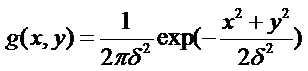求二阶偏导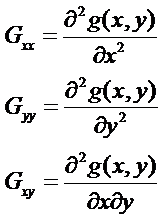对原图进行卷积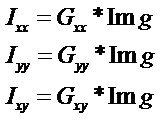构成Hessian矩阵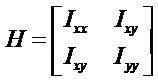2. 实现
% 提取Hessian特征值
function [hessianValue,Ixx,Ixy,Iyy] = edge_hessian(img)
[m n]=size(img);
w=4;
sigma=1.2;
[x y]=meshgrid(-w:w,-w:w);
% 高斯函数对应的二阶偏导
Dxx = 1/(-2*pi*sigma^4)*(1-x.^2/sigma^2)*exp(-(x.^2+y.^2)/(2*sigma^2));
Dyy = 1/(-2*pi*sigma^4)*(1-y.^2/sigma^2)*exp(-(x.^2+y.^2)/(2*sigma^2));
Dxy = 1/(2*pi*sigma^6)*(x.*y)*exp(-(x.^2+y.^2)/(2*sigma^2));

Ixx=imfilter(img,Dxx,'replicate');
Iyy=imfilter(img,Dyy,'replicate');
Ixy=imfilter(img,Dxy,'replicate');

hessianValue=[];
for i=1:m
for j=1:n
hessianValue(i,j) = Ixx(i,j)*Iyy(i,j) - Ixy(i,j)*Ixy(i,j);
end
end
end

Haar特征
1. 原理
Haar特征是一种反映图像的灰度变化的，像素分模块求差值的一种特征。常用于人脸识别中五官划分，例如：脸部的一些特征能由矩形模块差值特征简单的描述，如：眼睛要比脸颊颜色要深，鼻梁两侧比鼻梁颜色要深，嘴巴比周围颜色要深等。但矩形特征只对一些简单的图形结构，如边缘、线段较敏感，所以只能描述在特定方向（水平、垂直、对角）上有明显像素模块梯度变化的图像结构。
它分为三类：边缘特征、线性特征、中心特征和对角线特征。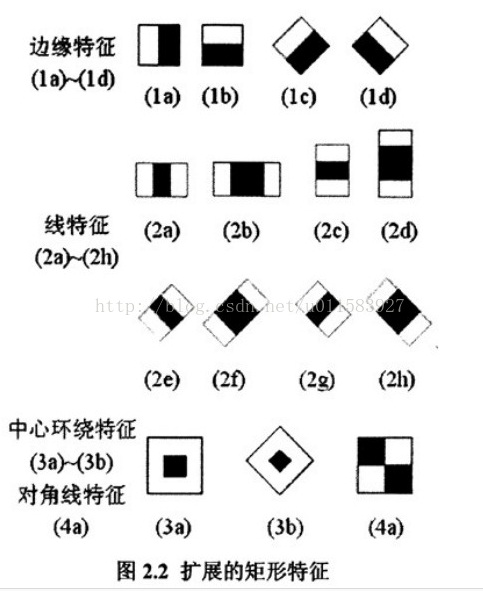模板特征值计算： 黑色矩形像素和 - 白色矩形像素和
注：一般通过积分图计算Haar特征，这样只需求一次积分图，就可以求出多种Haar特征，节省计算时间
积分图：主要思想是将图像从起点开始到各个点所形成的矩形区域像素之和作为一个数组的元素保存在内存中，当要计算某个区域的像素和时可以直接索引数组的元素，不用重新计算这个区域的像素和。示例如下：
[外链图片转存失败(img-9o0K5mMS-1562302602307)(https://img-my.csdn.net/uploads/201206/04/1338798979_5003.JPG)]
上图中，D块的像素和=II(4)+II(1)-II(2)-II(3) II表示积分图
2. 实现
这里我只实现了Haar特征的一种，其他的同理
% 提取Haar特征（中心为黑四周为白），思路如下：
% white+black = II(i+1,j+1)+II(i-2,j-1)-II(i-2,j+1)-II(i+1,j-2)
% black = img(i,j)
% harr = white-black = white+black-2*black
function [haar,hg,hgx,hgy] = edge_haar_center(img)
close all;
II = integralImage(img);% 求积分图
II = II(2:end,2:end);
height = size(II,1);
width = size(II,2);

total = II(4:height-1,4:width-1)+II(1:height-4,1:width-4)-II(1:height-4,4:width-1)-II(4:height-1,1:width-4);
black = img(3:height-2,3:width-2);
haar = (total - 2*black);
[hgx,hgy] = gradient(haar);
hg = sqrt(hgx.^2+hgy.^2);
end

参考资料
Canny边缘检测算法原理及其VC实现详解
Bilateral Filters（双边滤波算法）原理及实现
Hessian矩阵以及在图像中的应用
机器学习之Haar特征

展开全文hessian 双边滤波
• 确定图像中的物体边界一种方法是先检测每个像素和其直接淋雨状态已决定该像素是否确实处于一个物体边界上。具有这种特性像素被标记为边缘点。当图像各个像素灰度级用来反映各像素要求程度时，这种图像...
确定图像中的物体边界的一种方法是先检测每个像素和其直接淋雨的状态已决定该像素是否确实处于一个物体的边界上。具有这种特性的像素被标记为边缘点。当图像中各个像素的灰度级用来反映各像素要求的程度时，这种图像被称为边缘图像或边缘图。它也可以表示边缘点的位置而没有强弱程度的二值图像来表示。对边缘图像而不是幅度进行编码的图像叫做含方向边缘图。
一幅边缘图通常用边缘点勾画出各个物体的轮廓，单很少能形成图像分割所需要的闭合切连通的边界。因此需要对边缘点进行连接才能完成物体的检测过程。边缘点连接就是一个将邻近的边缘点连接起来，从而产生一条闭合的联通边界的过程。这个过程填补了因为噪声和阴影的影响所产生的间隙。--------引用：杨杰：数字图像处理及MATLAB实现

1.Roberts边缘算子
Roberts边缘检测算子是一种利用局部查分算子寻找边缘的算子。
2.Sobel边缘算子
两个卷积核形成了Sobel边缘算子。图像中的每个点都用这两个核做卷积。一个核对通常的垂直边缘响应最大而另一个对水平边缘响应最大。两个卷积的最大值作为该点的输出值。运算结果是一幅边缘幅度的图像
......理论省略，自行百度
%图像边缘检测
%加载图片并读取图片信息
srcImage=imread('E:\MatlabWorkSpace\实验三边缘检测\image.bmp');
srcImageInfo=imfinfo('E:\MatlabWorkSpace\实验三边缘检测\image.bmp');%图像为truecolor，首先转化为灰度图

%将图像转化为灰度图
grayImage=rgb2gray(srcImage);

%分别使用Roberts\Sobel\Prewitt\Canny算子进行边缘检测
imageRoberts=edge(grayImage,'roberts');
imageSobel=edge(grayImage,'sobel');
imagePrewitt=edge(grayImage,'prewitt');
imageCanny=edge(grayImage,'canny');

%显示检测图像
subplot(2,3,1);
imshow(grayImage);
title('原图像');

subplot(2,3,2);
imshow(imageRoberts);
title('roberts');

subplot(2,3,3);
imshow(imageSobel);
title('sobel');

subplot(2,3,4);
imshow(imagePrewitt);
title('prewitt');

subplot(2,3,5);
imshow(imageCanny);
title('canny');

展开全文• 几种常用的边缘检测算子： 边缘是图像的最重要的特征，。...边缘检测的基本思想： 利用边缘增强算子，突出图像的局部边缘； 然后定义像素的“边缘强度”，通过设置阈值的方法提取边缘点集。由于噪...
• 在常用一些边缘检测算子，Sobel常常形成不封闭区域，其他算子例如Laplace算子通常产生重响应。本章采用T型焊接焊缝图像进行分析，讨论了基于形态学处理焊缝边缘检测方法，该算法信噪比大且精度高。该算法...
• Matlab对图像的二值化以及边缘检测的轮廓提取方法canny算子和sobel算子源码DIY 方法1： BW = edge(I) 返回二值图像 BW，其中的值 1 对应于灰度或二值图像 I 函数找到边缘的位置，值 0 对应于其他位置。默认情况下...计算机视觉 图像处理 机器视觉
• 几种常用的边缘检测算子：边缘是图像的最重要的特征，。...边缘检测的基本思想：利用边缘增强算子，突出图像的局部边缘；然后定义像素的“边缘强度”，通过设置阈值的方法提取边缘点集。由于噪声和模糊的...
• 下面的Matlab GUI界面展示了基于小波变换的边缘检测的效果图。 2、基于小波变换的边缘检测 若需要相关代码可以在关注和订阅博主专栏之后，加博主QQ（2963033731）索取。 2.1 原始图像 2.2 边缘检测图像 2.3 简要...小波变换 图像处理 计算机视觉
• 实用标准文案 Matlab 图像锐化处理及边缘检测 本章要点 图像边缘锐化基本方法 微分运算 梯度锐化 边缘检测 6 1 图像边缘锐化基本方法 物体边缘是以图像局部特性不连续性形式出现 本质上边缘常意味着一个区域...
• 基于统计的图像边缘检测方法是计算机视觉中边缘检测的重要方法之一。提出了一种基于非参数变点统计分析的方向性边缘检测算法，该方法可以最小化对图像数字特征的先验信息的需求。深入讨论该算法在含有高斯噪声和椒盐...
• Matlab基于腐蚀和膨胀的边缘检测 文/天神  http://blog.sina.com.cn/s/blog_4a93ccea0100d25q.html 一.课题背景： 形态学运算只针对二值图像（二进制图像），并依据数学形态学（Mathermatical Morphogy...图像处理 函数
• matlab中sobel和kanny算子算法有多种实现方法，用简单可以看懂方式去编写 希望可以帮到大家
• 写本文的目的是记录自己做过的一些东西，深化理解，理清思路，便于回忆。本人处于学习阶段，欢迎指出不对的地方。 数学形态学之前文章解释过，...图像的边缘检测有多到数不清的方法，这里主要使用形态学的膨胀腐...
• Matlab 基于腐蚀和膨胀的边缘检测 文/天神 一.课题背景 形态学运算只针对二值图像二进制图像并依据数学形态学Mathermatical Morphogy集合论方法发展起来 图像处理方法起源于岩相对岩石结构定量描述工作在数字...
• 在Windows操作系统下的MATLAB2019b应用程序编写程序，待程序调试完成后，通过对多幅图像、加噪图像、去噪图像分别利用一阶微分、二阶微分算子进行边缘检测来探究Sobel算法、Log算法、Canny算法等三种常用的边缘...计算机视觉 图像处理
• 深度学习特别是全卷积神经网络的发展极大地促进了显著性检测的进步，但目前的方法对于目标边缘的处理还比较粗糙。为了获取更为精确的显著性目标区域，来自南开大学的研究人员利用显著性边缘和显著性目标间的互补性...
• 它的目标是找到一个最优的边缘检测算法，最优边缘检测的含义是：①好的检测- 算法能够尽可能多地标识出图像的实际边缘。②好的定位- 标识出的边缘要与实际图像的实际边缘尽可能接近。③最小响应- 图像的边缘...opencv
• 皂角花分割和边缘检测算法 MATLAB实现 图像处理和计算机视觉领域研究的发展 图像分割和边缘检测的问题在近二 十年得到了广泛的关注和长足的发展国内外很多研究人士提出了很多方法 在不同的领域取得了一定的成果 本...
• 引言：Matlab图像处理edge函数通常用于提取边缘。...BW = edge（I，method）使用方法指定的边缘检测算法检测图像I中的边缘 BW = edge（I，method，threshold）返回所有比阈值强边缘。 BW = edge...
• 实验经常需要测量一个物体的角度变化，如果开发一个图像处理的方法可以大大节省我们的时间，这次我们使用霍夫变换等进行物体夹角的预测。 任务 图像如下图所示 我们需要检测的夹角示意图为： 因此整个任务可以...计算机视觉 图像处理 霍夫变换
• 皂角花分割和边缘检测算法 MATLAB实现 图像处理和计算机视觉领域研究的发展图像分割和边缘检测的问题在近二 十年得到了广泛的关注和长足的发展国内外很多研究人士提出了很多方法 在不同的领域取得了一定的成果 本......

# matlab中边缘检测的方法matlab 订阅